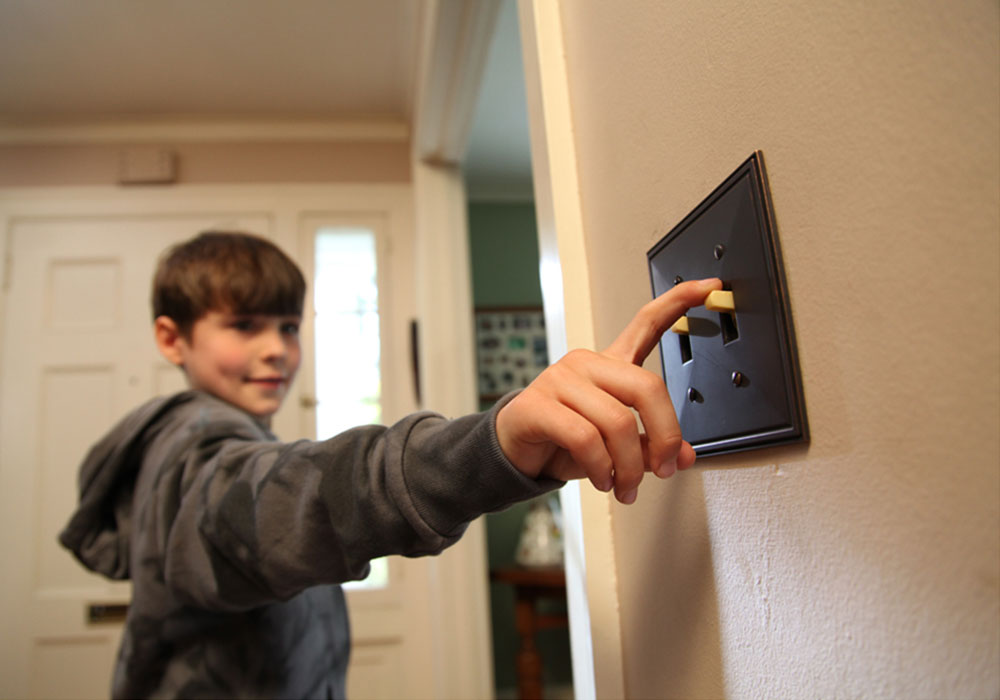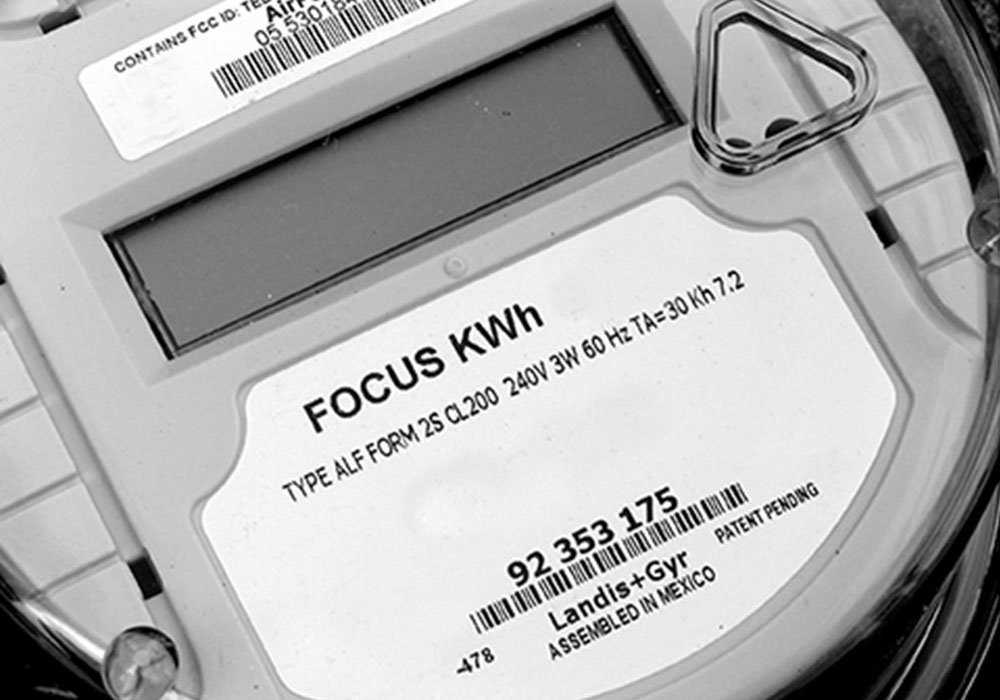You pay your bill every month, but what exactly are you paying for? How do we measure your energy use?

## Bill Breakdown

Cherryland works hard to make sure our member-owners pay competitive rates. If you are curious about the charges on your electric bill, check out our interactive bill demo below for explanations of charges.## What Is a Kilowatt Hour (kWh)?

Let’s start by defining a kilowatt hour. Different devices have different wattages: The average microwave, for example, is between 600 and 1,200 watts. To simplify things, let’s say you own a 1,000 watt microwave. If you cook your frozen meals for 5 minutes, and you eat 12 frozen meals a month, then you’ve used your microwave for an hour in total (you should also probably cut down on frozen meals).

Since your microwave is 1,000 watts, and you’ve used it for one hour, you’ve used 1 kilowatt hour for that month (just multiply the wattage by the number of hours used, then divide by 1000).

Here’s another example: If you run a 1,400-watt window AC unit for 8 hours a day, every day of the month, that will use up about 336 kWh for the month. Here’s the math: You used it for a total of 240 hours that month, because 8 hours a day x 30 days = 240. So, multiply the wattage (1,400) by the number of hours used (240), and then divide that by 1,000. Voila! Your AC unit cost you 336 kWh.

## Usage

Previous and Present: Most meters keep track of kilowatt hours used with a register that counts in ascending order, until it reaches 99,999 (at that point, it resets). So we just get your actual kWh used by subtracting “previous” from “present.”

Energy Charge: Simply, this is the charge based on how much energy you use.

Power Supply Cost Recovery (PSCR): In the energy industry, financial conditions surrounding power supply can change at the drop of a hat. So while we try to be as accurate as possible with our supply costs every year, sometimes we’re a little off. That’s when we need to adjust our PSCR, because as a non-profit, we’re not allowed to collect more or less than our actual cost of energy.